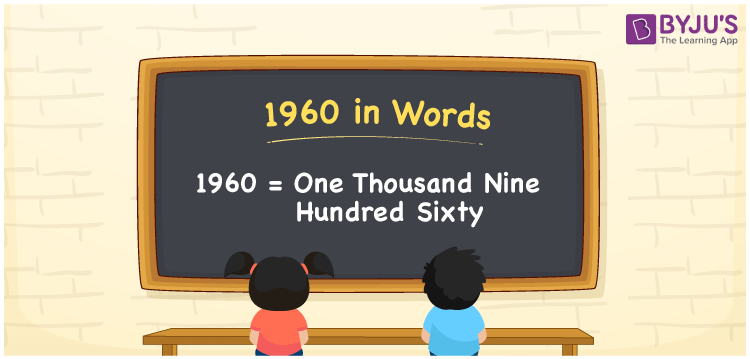# 1960 in words

1960 in words is written as One Thousand Nine Hundred and Sixty. 1960 represents the count or value. The article on Counting Numbers can give you an idea about count or counting. The number 1960 is used in expressions that relate to money, distance, length, year and others. Let us consider an example for 1960. “Neena’s mother was born in the year One Thousand Nine Hundred and Sixty”. Another example would be ”The distance between Bengaluru and Jaipur is nearly One Thousand Nine Hundred and Sixty kilometers.”

 1960 in words One Thousand Nine Hundred and Sixty One Thousand Nine Hundred and Sixty in Numbers 1960

## 1960 in English Words## How to Write 1960 in Words?

We can convert 1960 to words using a place value chart. The number 1960 has 4 digits, so let’s make a chart that shows the place value up to 4 digits.

 Thousands Hundreds Tens Ones 1 9 6 0

Thus, we can write the expanded form as:

1 × Thousand + 9 × Hundred + 6 × Ten + 0 × One

= 1 × 1000 + 9 × 100 + 6 × 10 + 0 × 1

= 1960

= One Thousand Nine Hundred and Sixty.

1960 is the natural number that is succeeded by 1959 and preceded by 1961.

1960 in words – One Thousand Nine Hundred and Sixty.

Is 1960 an odd number? – No.

Is 1960 an even number? – Yes.

Is 1960 a perfect square number? – No.

Is 1960 a perfect cube number? – No.

Is 1960 a prime number? – No

Is 1960 a composite number? – Yes

## Solved Example

1. Write the number 1960 in expanded form

Solution: 1 × 1000 + 9 × 100 + 6 × 10 + 0 × 1

We can write 1960 = 1000 + 900 +60 + 0

= 1 × 1000 + 9 × 100 + 6 × 10 + 0 × 1

## Frequently Asked Questions on 1960 in words

Q1

### How to write 1960 in words?

1960 in words is written as One Thousand Nine Hundred and Sixty.
Q2

### Is 1960 a perfect square number?

No. 1960 is not a perfect square number.
Q3

### Is 1960 a prime number?

No. 1960 is not a prime number.